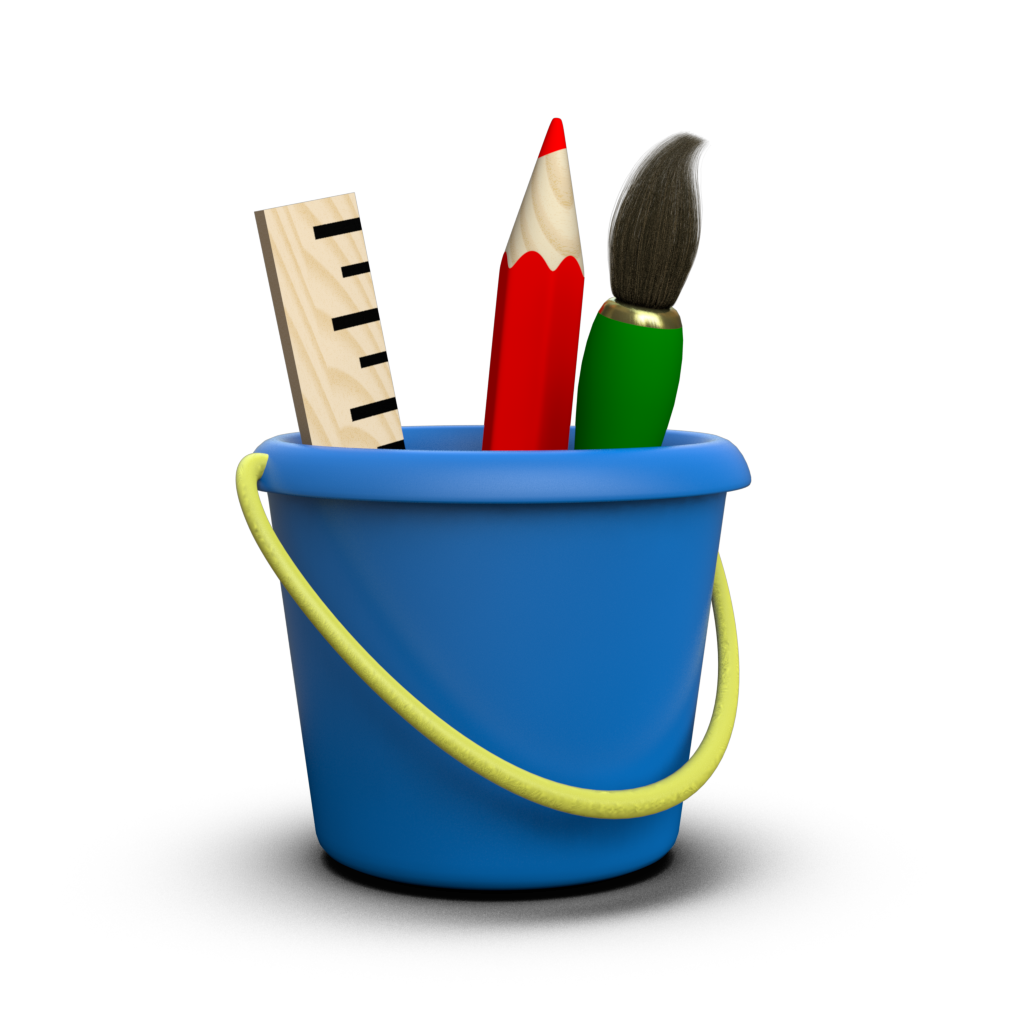# Null Data¶

“Never tell a lie” is an integral part of The Toyplot Ethos - and Toyplot’s handling of null data is one of the ways that we honor it. Consider the following data, in which several datums contain floating-point [NaN](https://en.wikipedia.org/wiki/NaN) values:

:

import numpy
x = numpy.linspace(0, 2 * numpy.pi)
y = numpy.sin(x)
y[6:20] = numpy.nan


When we plot this data, Toyplot carefully takes the NaN values into account:

:

import toyplot.data
toyplot.plot(x, y, ymax=1, marker="o", width=600, height=300);


Note that the Y axis domain reflects the lack of data where there are NaN values, and there are no markers for the NaN datams. Note too that the plot has been broken into two segments - drawing a segment through the NaN region might mislead viewers about the shape of the curve, while breaking the plot unambiguously communicates the absence of data.

Of course NaN values can only be used with floating-point arrays, so there must be alternate ways to represent null values for other data types such as integers. To address this, Toyplot uses masked arrays for all its internal data structures, and accepts masked arrays for its inputs, allowing you to define null values in your data explicitly:

:

numpy.random.seed(1234)
y = numpy.ma.array(numpy.random.choice(numpy.arange(3, 10), size=50))
toyplot.bars(y, width=600, height=300);


You might feel that masking null values in the above example is needlessly complex, when a special value of “zero” could accomplish the same thing. But consider what happens if there is more than one series:

:

magnitudes = numpy.ma.column_stack((
numpy.random.choice(numpy.arange(5, 10), size=50),
numpy.random.choice(numpy.arange(5, 10), size=50),
))
magnitudes[5:15,0] = 0
toyplot.bars(magnitudes, width=600, height=300);


The position of the bars in the second series suggest that the null values in the first series actually have a value of zero, when in reality we want to communicate that they have no value at all. Contrast this with what Toyplot produces when you correctly mark the values as null instead of zero:

:

magnitudes[5:15,0] = numpy.ma.masked
toyplot.bars(magnitudes, width=600, height=300);


Toyplot now removes entire observations that contain null values. Note that this behavior is dictated by the structure of the visualization - because we use stacked bars to represent data where the sum of the magnitudes is significant, a null anywhere in that sum makes the entire sum null and void.

This is not the case for all visualizations, of course. Consider what happens when rendering a set of bar boundaries, rather than a set of bar magnitudes:

:

observations = numpy.random.normal(size=(50, 50))
boundaries = numpy.ma.column_stack((
numpy.min(observations, axis=1),
numpy.median(observations, axis=1),
numpy.max(observations, axis=1),
))

toyplot.bars(boundaries, baseline=None, width=600, height=300);


Now, suppose that some of the lower boundaries in the plot are null:

:

boundaries[5:10, 0] = numpy.ma.masked
toyplot.bars(boundaries, baseline=None, width=600, height=300);


In this case, the position of each bar is defined by two boundaries. Only those bars with missing boundaries are left out - the adjacent bars are still visible because they are still unambigously well-defined. The same would be true if some of the top boundary values were null:

:

boundaries[40:45, 2] = numpy.ma.masked
toyplot.bars(boundaries, baseline=None, width=600, height=300);


Finally, as you might imagine, null values in the middle boundary affect both sets of adjacent bars:

:

boundaries[20:30, 1] = numpy.ma.masked
toyplot.bars(boundaries, baseline=None, width=600, height=300);


Of course, these behaviors extended to other plot types too:

:

toyplot.fill(magnitudes, baseline="stacked", width=600, height=300);

:

toyplot.fill(boundaries, width=600, height=300);


Finally, a special-case worth mentioning is Toyplot table visualizations, which can make an explicit distinction between null and NaN values:

:

data = toyplot.data.Table()
data["a"] = numpy.random.random(11)
data["b"] = numpy.random.random(11)

:

canvas, table = toyplot.table(data, width=300, height=350)